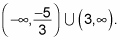##### Pre-Calculus For Dummies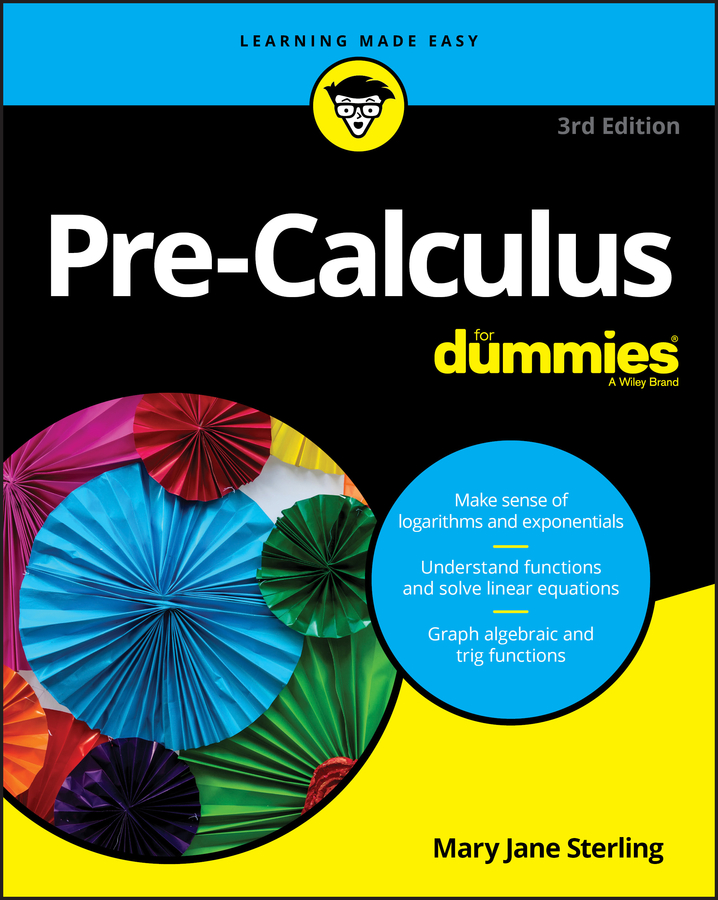You can use interval notation to express where a set of solutions begins and where it ends. Interval notation is a common way to express the solution set to an inequality, and it’s important because it’s how you express solution sets in calculus. Most pre-calculus books and some pre-calculus teachers now require all sets to be written in interval notation.

The easiest way to find interval notation is to first draw a graph on a number line as a visual representation of what’s going on in the interval.

If the endpoint of the interval isn’t included in the solution (for < or >), the interval is called an open interval. You show it on the graph with an open circle at the point and by using parentheses in notation. If the endpoint is included in the solution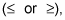the interval is called a closed interval, which you show on the graph with a filled-in circle at the point and by using square brackets in notation.

For example, the solution set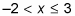is shown here.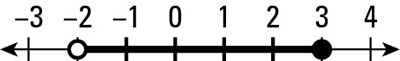Note: You can rewrite this solution set as an and statement: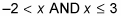In interval notation, you write this solution as (–2, 3].

The bottom line: Both of these inequalities have to be true at the same time.

You can also graph or statements (also known as disjoint sets because the solutions don’t overlap). Or statements are two different inequalities where one or the other is true. For example, the next figure shows the graph of x < –4 OR x > –2.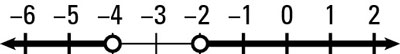Writing the set for this figure in interval notation can be confusing. x can belong to two different intervals, but because the intervals don’t overlap, you have to write them separately:

• The first interval is x < –4. This interval includes all numbers between negative infinity and –4. Because negative infinity isn’t a real number, you use an open interval to represent it. So in interval notation, you write this part of the set as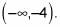• The second interval is x > –2. This set is all numbers between –2 and positive infinity, so you write it as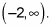You describe the whole set as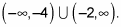The symbol in between the two sets is the union symbol and means that the solution can belong to either interval.

When you’re solving an absolute-value inequality that’s greater than a number, you write your solutions as or statements. Take a look at the following example: |3x – 2| > 7. You can rewrite this inequality as 3x – 2 > 7 OR 3x – 2 < –7. You have two solutions: x > 3 or x < –5/3.

In interval notation, this solution is